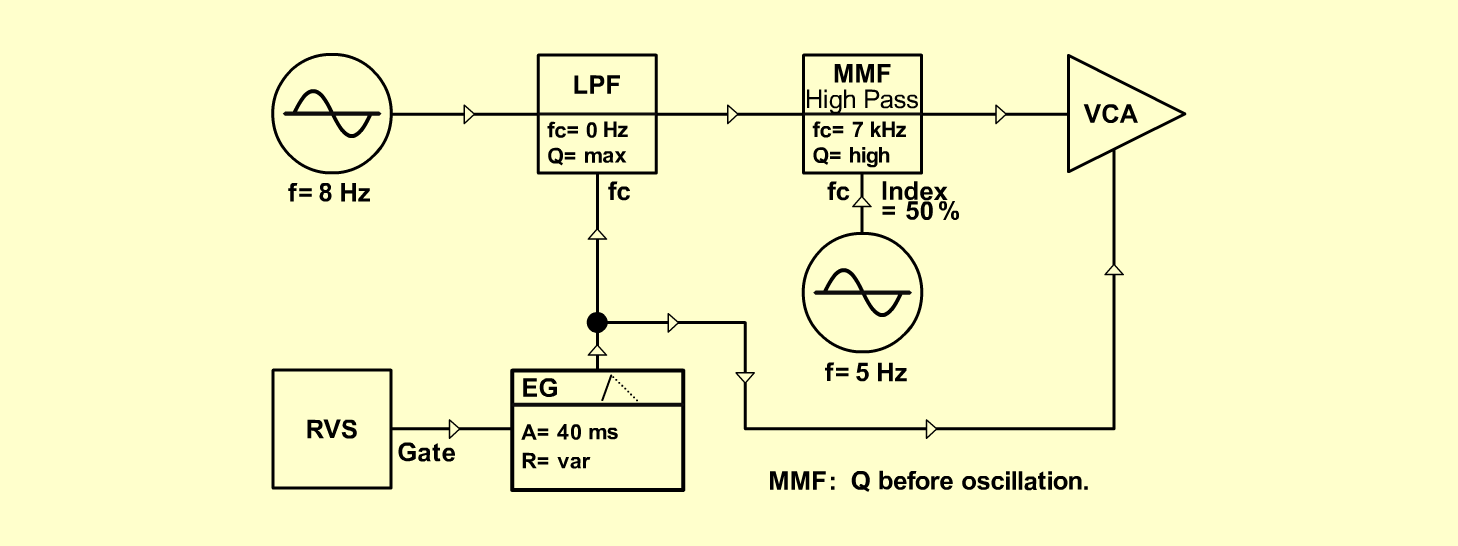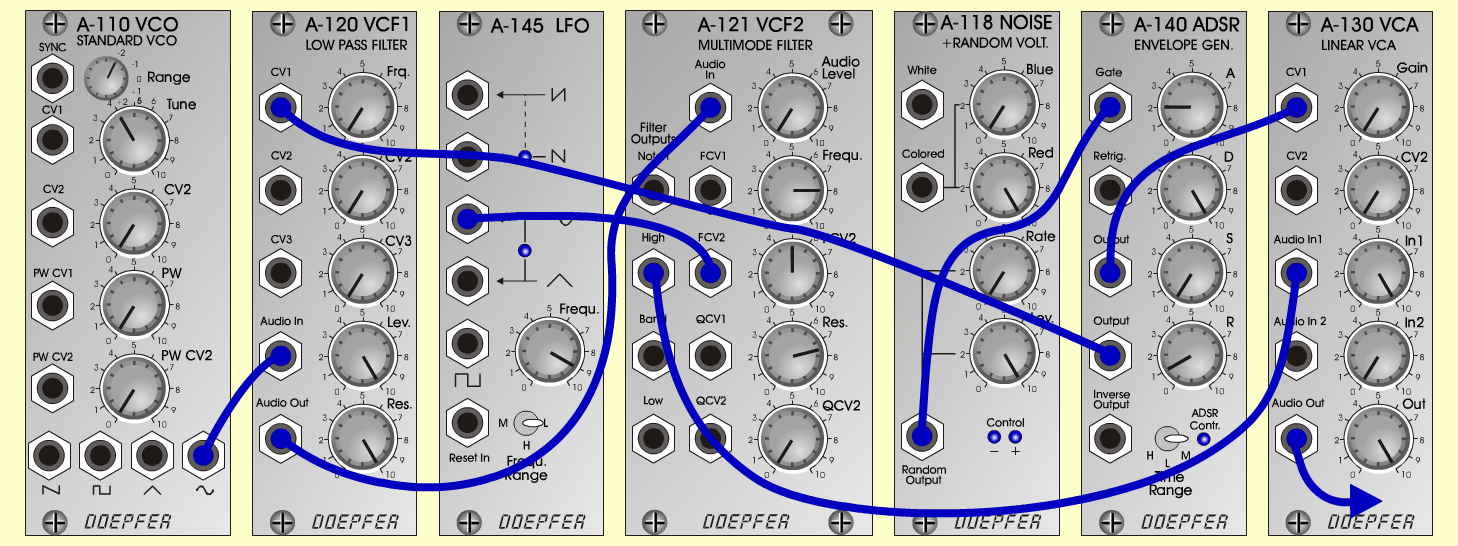TwitterN a t u r eD o e p f e r A - 100 Connections: Settings: A-110 (Sine) <=> A-120 (Audio In)   A-120 (Audio Out) <=> A-121 (Audio In)   A-121 (High) <=> A-131 (Audio In)   A-118 (Random Output) <=> A-140 (Gate)   A-140 (Output 1) <=> A-131 (CV1)   A-140 (Output 2) <=> A-120 (CV1)   A-145 (Sine) <=> A-121 (CV2)   A-131 (Audio Out) <=> Amplifier A-110 (Range=-2, Tune=4)   A-120 (Freq=0, Lev=10, Res=10)   A-145 (Freq = 9, Range = L)   A-121 (Freq=8, FCV2 = 5, Res=7.5)   A-118 (Red=10, Rate=0, Level=10)   A-140 (A=2, D=10, S=0, R=1, Range = M)   A-130 Exp (Gain=0, In 1=10, Out=10) Notes: Change pitch and timbre with        A-110 Range-pot (frequency) and       A-120 Freq-pot. Josef MuellerSound sample Twitter